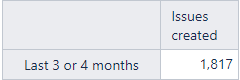# Use a Case statment returning Aggregate

Hello,

I am trying to use a Case statment and returning an Aggregate of dates, but it doesn’t work as I expected. My calculated member is like this:

CASE WHEN Day(Now()) <= 7 THEN
Aggregate(
[Time].[Month].DateMembersBetween(‘4 months ago’, ‘today’)
)
ELSE
Aggregate(
[Time].[Month].DateMembersBetween(‘3 months ago’, ‘today’)
)
END

The name of the calculated member is “Last 3 or 4 months” and the result is like this:As you can see it doesn’t show the ‘+’, to see how many issues where created each month, it only shows the total of the aggregation.

How can I do this calculated member to show the Aggregate with the ‘+’ symbol?

Thank you.

Best regards.

Alex.

Hello Alex,

Application is having some difficulties distinguishing whether anything that is not plain Aggregate is an aggregation. Therefore your formula is considered case selection, and you cannot see the constituting members.

The solution lies in bringing the Aggregate to the top level of the formula and redesigning the case selection.
As the difference is only in one letter/digit - let us put IIF condition there.

``````Aggregate(
[Time].[Month].DateMembersBetween(
Format(
IIF(Day(Now())<=7,
4,
3),
'#') ||' months ago',
'today')
)
``````

Now calculated member is a clear Aggregate, and you may expand it to see underlying members.

Kind regards,
Oskars / support@eazybi.com

Hello,

It worked perfectly.

Thenks.

Alex.